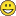# Labeling the sequence using concatenation

593
4
10-27-2018 09:03 PMNew Contributor II

Hi again..
sorry for asking so many questionsi just found interest way working arcgis with python..
btw im new to this way of working so im sorry if the question sometime hard to understand..

so here is my question..
i have feature point data with 3 fields in table attribute (objectID, shape and sequence)..
i want to add another field lets say called "name_sequence)..

in the name_sequence field i want to add text which have format "x+yyy", ("x", "+", "yyy")

so it become like this

0+000, 0+100, 0+200, .... 0+900

the point after +900 it will add "x+1" so in data no 11 it'll show 1+000 after that they will have same sequence as i mention above..
furthermore, after i searching the code i found we can use range function and concat function to do that..
so i come with the code like this..

``````import arcpy

new_field = 'Seq_Name' #Change
fc = r'directory' #Change

for i = 1 to 10
a = i
b = i*100
srt_join = concat(srt(a),"+", srt(b))
end for ‍‍‍‍‍‍‍‍‍‍‍‍``````

i know it might be totally wrong code or it is not complete yet.

so i want to know what should i add for this code?

or do you have another way to do this without arcpy?

and i want know new understanding about python's things..

for your understanding i attach the result of my data which i working in excel..

Tags (4)
1 Solution

Accepted SolutionsbyEsri Esteemed Contributor

I didn't test the code, but I assume you could use something like this (untested):

``````def main():
import arcpy
fc = r'Drive:\Path\To\Source\FC'
fld_seq = 'SequenceLbl'

# start update cursor to update the values in the new field
flds = (fld_seq)
cnt = 0
with arcpy.da.UpdateCursor(fc, flds) as curs:
for row in curs:
cnt += 1
km, hect = divmod(cnt, 10)
hect_txt = "%03d" % (hect * 100,)
lbl = "{}+{}".format(km, hect_txt)
print cnt, hect_txt
curs.updateRow((lbl, ))

if len(arcpy.ListFields(tbl, fld_name)) == 0:
arcpy.AddField_management(tbl, fld_name, fld_type, None, None, fld_length)

if __name__ == '__main__':
main()‍‍‍‍‍‍‍‍‍‍‍‍‍‍‍‍‍‍‍‍‍‍‍‍‍‍‍``````

When I run some test code like shown below:

``````def main():
cnt = 0
for i in range(25):
cnt += 1
km, hect = divmod(cnt, 10)
hect_txt = "%03d" % (hect * 100,)
lbl = "{}+{}".format(km, hect_txt)
print("{}:  {}".format(cnt, lbl))

if __name__ == '__main__':
main()‍‍‍‍‍‍‍‍‍‍‍``````

... the output is:

``````1:  0+100
2:  0+200
3:  0+300
4:  0+400
5:  0+500
6:  0+600
7:  0+700
8:  0+800
9:  0+900
10:  1+000
11:  1+100
12:  1+200
13:  1+300
14:  1+400
15:  1+500
16:  1+600
17:  1+700
18:  1+800
19:  1+900
20:  2+000
21:  2+100
22:  2+200
23:  2+300
24:  2+400
25:  2+500‍‍‍‍‍‍‍‍‍‍‍‍‍‍‍‍‍‍‍‍‍‍‍‍‍``````
4 RepliesbyMVP Esteemed Contributor

unless you want to get into updatecursors or insertcursors and you seem to be using excel a lot,

why not have a look at a quick script call to this do bring in your spreadsheet directlybyEsri Esteemed Contributor

I didn't test the code, but I assume you could use something like this (untested):

``````def main():
import arcpy
fc = r'Drive:\Path\To\Source\FC'
fld_seq = 'SequenceLbl'

# start update cursor to update the values in the new field
flds = (fld_seq)
cnt = 0
with arcpy.da.UpdateCursor(fc, flds) as curs:
for row in curs:
cnt += 1
km, hect = divmod(cnt, 10)
hect_txt = "%03d" % (hect * 100,)
lbl = "{}+{}".format(km, hect_txt)
print cnt, hect_txt
curs.updateRow((lbl, ))

if len(arcpy.ListFields(tbl, fld_name)) == 0:
arcpy.AddField_management(tbl, fld_name, fld_type, None, None, fld_length)

if __name__ == '__main__':
main()‍‍‍‍‍‍‍‍‍‍‍‍‍‍‍‍‍‍‍‍‍‍‍‍‍‍‍``````

When I run some test code like shown below:

``````def main():
cnt = 0
for i in range(25):
cnt += 1
km, hect = divmod(cnt, 10)
hect_txt = "%03d" % (hect * 100,)
lbl = "{}+{}".format(km, hect_txt)
print("{}:  {}".format(cnt, lbl))

if __name__ == '__main__':
main()‍‍‍‍‍‍‍‍‍‍‍``````

... the output is:

``````1:  0+100
2:  0+200
3:  0+300
4:  0+400
5:  0+500
6:  0+600
7:  0+700
8:  0+800
9:  0+900
10:  1+000
11:  1+100
12:  1+200
13:  1+300
14:  1+400
15:  1+500
16:  1+600
17:  1+700
18:  1+800
19:  1+900
20:  2+000
21:  2+100
22:  2+200
23:  2+300
24:  2+400
25:  2+500‍‍‍‍‍‍‍‍‍‍‍‍‍‍‍‍‍‍‍‍‍‍‍‍‍``````New Contributor II

solved..

thanks Mr BakkerbyEsri Esteemed Contributor